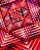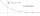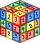# Q of GP

Calculate quotient of geometric progression if a1=5 a a1+a2=12.

Correct result:

q =  1.4

#### Solution:We would be pleased if you find an error in the word problem, spelling mistakes, or inaccuracies and send it to us. Thank you!## Next similar math problems:

• Five elementThe geometric sequence is given by quotient q = 1/2 and the sum of the first six members S6 = 63. Find the fifth element a5.
• Geometric progression 2There is geometric sequence with a1=5.7 and quotient q=-2.5. Calculate a17.
• Sum of GP membersDetermine the sum of the GP 30, 6, 1.2, to 5 terms. What is the sum of all terms (to infinity)?
• QuotientDetermine the quotient and the second member of the geometric progression where a3=10, a1+a2=-1,6 a1-a2=2,4.
• GP membersThe geometric sequence has 10 members. The last two members are 2 and -1. Which member is -1/16?
• Find the 19Find the 1st term of the GP ___, -6, 18, -54.
• GP - 8 itemsDetermine the first eight members of a geometric progression if a9=512, q=2
• Difference AP 4Calculate the difference of the AP if a1 = 0.5, a2 + a3 = -1.1
• What is 10What is the 5th term, if the 8th term is 80 and common ratio r =1/2?
• Geometric seqFind the third member of geometric progression if a1 + a2 = 36 and a1 + a3 = 90. Calculate its quotient.
• GP - three membersThe second and third of a geometric progression are 24 and 12(c+1) respectively, given that the sum of the first three terms of progression is 76 determine value of c
• If the 3If the 6th term of a GP is 4 and the 10th is 4/81, find common ratio r.
• What are 2What are the two more terms of the GP a, ax, ax2, ax3, __, __?
• Tenth memberCalculate the tenth member of geometric sequence when given: a1=1/2 and q=2
• Geometric progressionIn geometric progression, a1 = 7, q = 5. Find the condition for n to sum first n members is: sn≤217.
• Find next memberFind x if the numbers from a GP 7, 49, x.
• CoefficientDetermine the coefficient of this sequence: 7.2; 2.4; 0.8